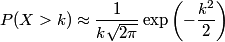# Variation in male and female Olympic performance

Isabel Lugo posted an interesting article today called Variance in Olympic events in which she speculates about the variance in male versus female athletic performance.

… it may be the case that the difference between the very best men and the very best women in physical feats (say, times in some sort of race, because these are the most easily quantified) is larger than the difference between the average man and the average woman, because there could be more variance among men than women.

I did a few back-of-the-envelope calculations to explore this possibility. Let X represent female athletic performance and Y male athletic performance in some context. Assume X and Y are normally distributed and that we have rescaled so that X has mean 0 and standard deviation 1. (I know nothing about the statistics of athletic performance. This is just a rough exercise inspired by Isabel Lugo’s question.) For this post, I will assume equal numbers of men and women are interested in a given sport. My next post looks at what happens when abilities are equal but more men than women are interested in a given sport.

First, suppose men and women have equal average performance but that men have standard deviation σ > 1. Then a man who just makes the cutoff of n standard deviations above mean has performance nσ and a woman who just makes the analogous cutoff has performance n. Then the ratio of their performance is σ for any value of n. At every percentile, the ratio of male to female performance would be the same. The difference in performance, n(σ – 1), does increase as you look at more elite athletes, i.e. increasing values of n, but not by much. The difference would only be larger by 25% when looking at 5-sigma athletes rather than 4-sigma athletes even though the former is over 100 times more exclusive.

What if in some context male and female performance both had variance 1 but had different means? Say the mean for men is μ > 0 and the mean for women is 0. Then the performance for a man n standard deviations from the mean for men would be μ + n and the performance for a woman n standard deviations away from the mean for women would be n. The difference would remain constant at all levels of performance, but the ratio of performance levels would tend toward 1 as n increases, that is, as you look at more and more elite athletes.

Next look at a different question. In either of the above situations, what proportion of the best athletes will be male? I will show that the odds of a top athlete being male increase exponentially as your definition of “top” increases.

For a given level of performance k, we will look at P(Y > k)/P(X > k), the ratio of the proportion of men at that level to the proportion of women at that level. The probability that a woman has performance greater than k is given by the approximationNow suppose Y has mean 0 but standard deviation σ > 1. Then the odds in favor of someone with performance level greater than k being male equalswhich increases exponentially as k increases, i.e. as we look at higher levels of performance. (By symmetry, this would also mean that the odds of a poor performer being male would increase as you looked at worse and worse performers.) To plug in some particular numbers, suppose the standard deviation for men is 1.5 and we had a group of people with performance 2 or greater. The odds in favor of someone in that group being male would be almost 4 to 1. But if we looked in a group with performance 5 or greater, the odds in favor of someone being male would be 322 to 1.

Next suppose Y has mean μ > 0 but standard deviation 1. Then the odds of a top performer being male areThis also increases exponentially as k increases. Again to put in some specific numbers, assume μ = 0.5 and look at performance levels of 2 and 5. The odds in favor of someone with performance level at least 2 being male are about 3.2 to 1. The corresponding odds for a group with performance level at least 5 are about 12 to 1.